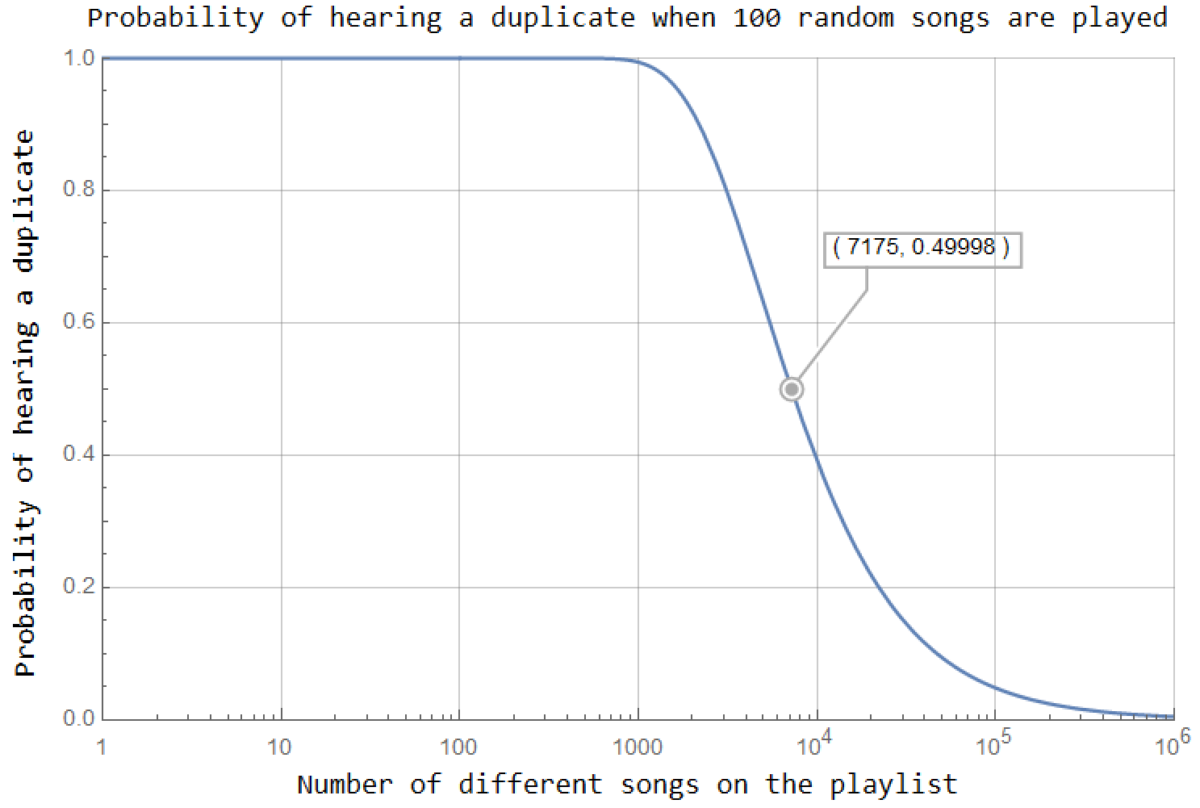# Elf musicThis holiday-themed Riddler problem is about probability:

In Santa’s workshop, elves make toys during a shift each day. On the overhead radio, Christmas music plays, with a program randomly selecting songs from a large playlist.

During any given shift, the elves hear 100 songs. A cranky elf named Cranky has taken to throwing snowballs at everyone if he hears the same song twice. This has happened during about half of the shifts. One day, a mathematically inclined elf named Mathy tires of Cranky’s sodden outbursts. So Mathy decides to use what he knows to figure out how large Santa’s playlist actually is.

Help Mathy out: How large is Santa’s playlist?

Here is my solution:
[Show Solution]

## 5 thoughts on “Elf music”

1.Dave Moran says:

I got the same answer, but I was surprised it was so high.

2.Joe Schoneman says:

I arrived at this from the opposite end. After the first song plays, there is an $\frac{N – 1}{N}$ probability of a duplicate song playing; after the second, the probability of a duplicate song playing is $\frac{N-2}{N}$ and so on. Once $m$ songs have played, the probability of a duplicate song having played is thus $P_{dup} = \Pi_{k=0}^{m-1} \frac{N – k}{N}$. This doesn’t account for the fact that Grumpy throws a snowball at the radio if a duplicate plays, but that doesn’t matter for our purposes here.

It turns out that $\Pi_{k=0}^{m-1}(N-k)$ is defined as the “falling factorial” $(N)_m = N(N – 1)(N – 2) \cdots (N – (m – 1)$, which can be expressed in terms of the Gamma function as $(N)_k = \dfrac{\Gamma[N + 1]}{\Gamma[N – (m – 1)]}$. So our duplicate probability becomes $P_{dup} = \left(\frac{1}{N}\right)^m \dfrac{\Gamma[N + 1]}{\Gamma[N – m + 1]}$.

The nice thing about the Gamma function is that it retains its validity even for $N < m$ and will properly calculate the resultant zero probability of this case. If we want to simplify further, though, we can swap in factorials to end up with $P_{dup} = \left(\frac{1}{N}\right)^m \dfrac{N!}{(N – m)!}$ for $N \geq m$, which aligns with the complement of your result.

The slight downside here is that I had to rely on multi-precision arithmetic to actually compute these quantities (in Python) whereas a direct numerical evaluation of the product series gave the correct answer quickly and painlessly. Can't win them all.

3.Josh Jordan says:

My solution is like Laurent’s. I wrote code in Go to find the answer and run simulations to check it.

4.Steven Pratt says:

This is basically a twist on the oft-misunderstood birthday paradox, where it is mathematically proven that a random selection of 50 people has a 98%+ chance of having two people who share a birthday)

Since this requires such large numbers, I’ll pull up a spreadsheet and show my work later today or tomorrow. I think to solve this I’ll be adding the log10 of lots of numbers…

But the answer is x, where (x^100)/(x! / (x-100)!) = 0.5

1.Mike Strong says:

This is exactly how I attacked the problem. Then, I used Stirling’s approximation to get rid of the factorials, and did some log manipulations to reduced it to a equation that was easily solved using a calculator and trial and error.